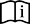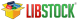Trigonometry Library

The mikroC PRO for PIC implements fundamental trigonometry functions. These functions are implemented as look-up tables. Trigonometry functions are implemented in integer format in order to save memory.

sinE3

Prototype int sinE3(unsigned angle_deg); The function returns the sine of input parameter. The function calculates sine multiplied by 1000 and rounded to the nearest integer: result = round(sin(angle_deg)*1000) Parameters : angle_deg: input angle in degreesNote : Return value range: -1000..1000 Nothing. int res; ... res = sinE3(45); // result is 707

cosE3

Prototype int cosE3(unsigned angle_deg); The function returns the cosine of input parameter. The function calculates cosine multiplied by 1000 and rounded to the nearest integer: result = round(cos(angle_deg)*1000) Parameters : angle_deg: input angle in degreesNote : Return value range: -1000..1000. Nothing. int res; ... res = cosE3(196); // result is -961
Find them on